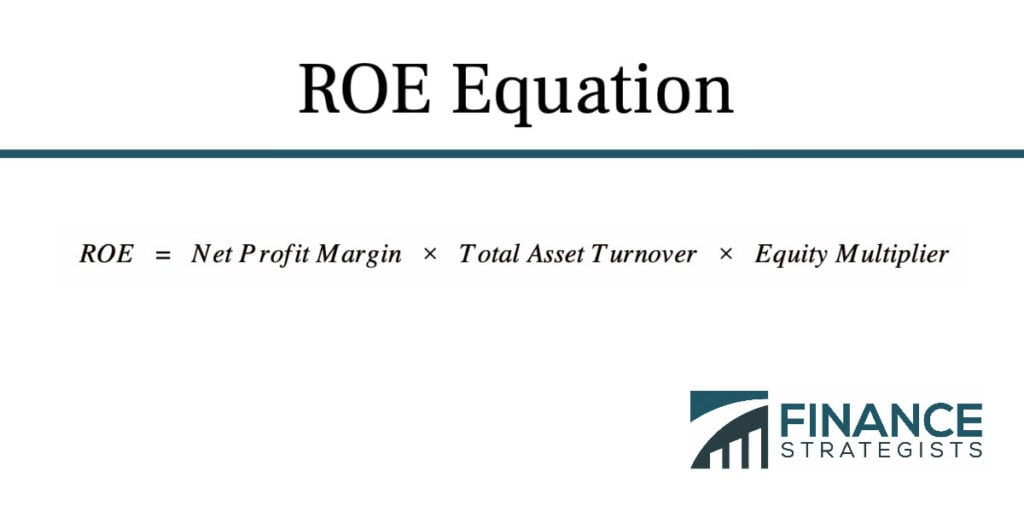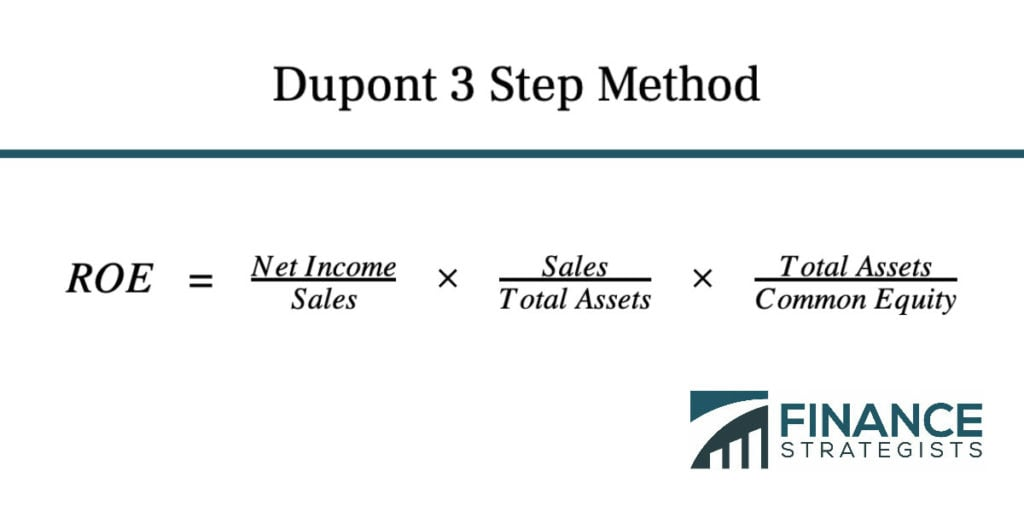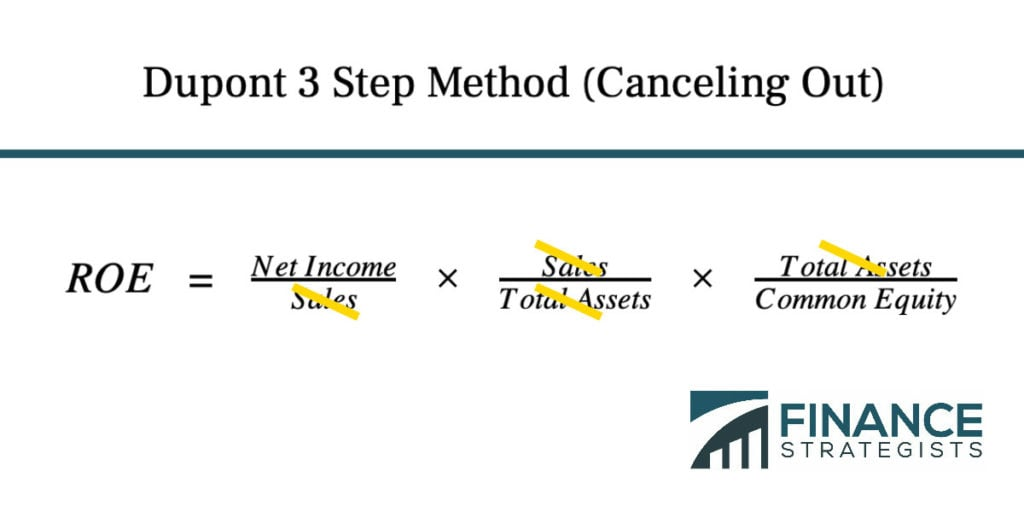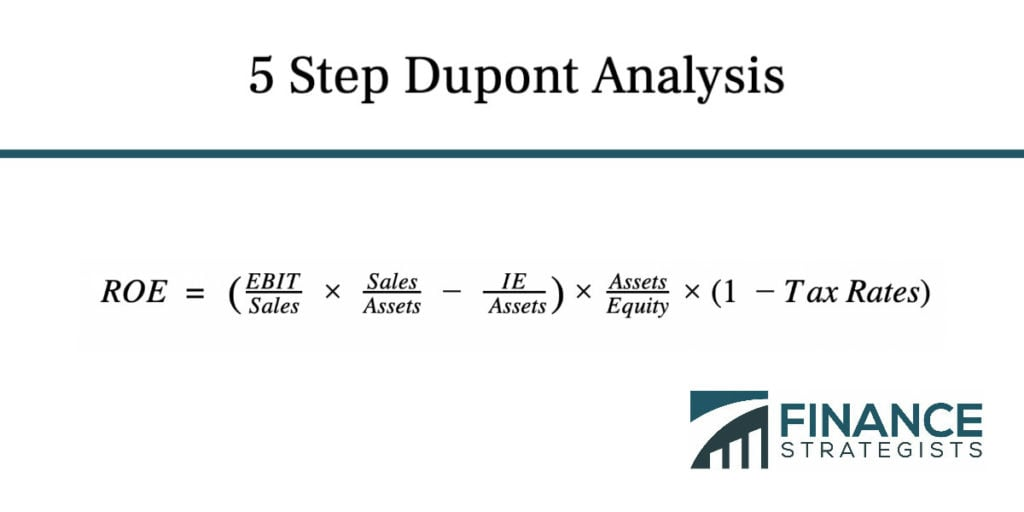# DuPont Analysis### Written byTrue Tamplin, BSc, CEPF® | Reviewed by Editorial Team

Updated on January 05, 2023

## What Is DuPont Analysis?

The DuPont Analysis Formula is an alternate way to calculate and deconstruct ROE (Return on Equity) in order to get a better understanding of the underlying factors behind a company's ROE. This allows analysts to understand where a company is strong and where it is weak when it comes to generating profitability.

The DuPont Analysis gets its name from the company that pioneered the detailed model in the 1920s, the DuPont Corporation.

Whereas ROE relies on a simple calculation of net income divided by shareholder equity in order to evaluate a company's performance, the DuPont formula goes deeper, focusing on three crucial categories of ROE:

1. Operating efficiency
2. Asset efficiency
3. Leverage

## DuPont Method

The DuPont Method has three main components.

1. The first is operating efficiency, which is measured by net profit margin. This shows how much money in net sales is generated per every dollar in expenses. Net profit margin is calculated by dividing net profit, also known as net income, by revenue.
2. The second component, asset efficiency, is measured by total asset turnover. This determines how many dollars of total revenue a company generates per dollar in assets. Total asset turnover is calculated by dividing a company's revenue by the total assets that it has on hand.
3. The third component is financial leverage, determined by the equity multiplier. Unlike the first two components, which directly evaluate a company's operations, financial leverage assesses how well a company is using debt, a key driver of ROE, to finance those operations.
The equity multiplier is calculated by dividing a company's assets by its equity.

## DuPont Formula

The simplest DuPont formula, the three-step method, is done by simply multiplying the three determinants of three main components--net profit margin, total asset turnover, and equity multiplier--to determine the ROE.When broken down into the calculations for the different components, the DuPont three-step method formula looks like this:This is simply a more detailed version of the basic ROE calculation, which can still be reached by simply cross-canceling out the sales and total assets metrics.## DuPont ROE

As can be seen above, the DuPont method is just a more complicated way to calculate ROE. However, it can be a more effective tool for investors and analysts because it goes into deeper detail of what is driving a company's ROE.

For instance, if a company's assets are losing value, it may still maintain a healthy ROE by accumulating more debt, which could possibly be a warning sign to investors depending on how much debt is accumulated.

On the other hand, if a company is relying more on its profit margin to generate its ROE without accumulating much debt, an investor may be more inclined to invest in that company because there is lower risk that the company will take on too much debt.

When taking the DuPont method into account, basic ROE can be far less misleading, especially on the issue of a company's debt.

## DuPont Framework

There is a second, slightly more detailed version of the DuPont method called the five-step DuPont. This allows investors to see whether a company is propping up its ROE through accumulating debt while suffering from a low profit margin and/or depreciating assets.

The individual calculations for determining asset efficiency and financial leverage remains the same, but the net profit margin formula, used to calculate operational efficiency, changes to better show a company's earnings before interest and taxes, or EBIT.

The EBIT is calculated by subtracting a company's earnings before taxes (EBT) by its interest expense (IE). The rest of the equation is then multiplied by one minus the tax rate in order to determine the tax hit on a company.

When spelled out, the five-step DuPont equation looks like this:## DuPont Equation

Both the three-step and five-step DuPont equations allow investors a more thorough glimpse of the year-over-year changes in a company. These equations allow them to better determine whether that company is headed in the right direction in ways that are impossible to examine by looking at ROE alone.

Additionally, the DuPont model uses gross asset value, which ignores depreciation, instead of net asset value. This means managers are incentivized to get the most out of the useful life of the assets they already have on hand instead of borrowing more and investing in new assets.

## DuPont Ratio

Even though the DuPont method goes above and beyond a simple ROE calculation to give investors a better look at a company's operations, profitability, asset value and debt accumulation, it still has limitations.

When considering a company's ROE, even with the DuPont formula, investors should keep in mind the average ROE for that company's industry, as well as rival companies' ROEs, and determine where that ROE stands in comparison.

The DuPont ratio alone cannot accomplish this.

## DuPont Model (DuPont Analysis) FAQs

### What is DuPont Analysis?

The DuPont Analysis Formula is an alternate way to calculate and deconstruct ROE (Return on Equity) in order to get a better understanding of the underlying factors behind a company’s ROE.

### Why would an investor look at a DuPont ROE formula?

The DuPont method is key because Return on Equity is a major component of what an investor looks at when evaluating the performance of various investments.

### What does the DuPont Analysis show?

The DuPont Analysis allows analysts to understand where a company is strong and where it is weak when it comes to generating profitability.

### Who invented the DuPont Analysis?

The DuPont Analysis got its name from the company that pioneered the detailed model in the 1920s, the DuPont Corporation.

### What are the three components of the DuPont Analysis?

The components that make up the DuPont Analysis are operating efficiency, asset efficiency, and leverage.